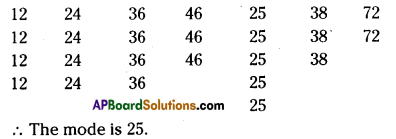AP State Syllabus AP Board 7th Class Maths Solutions Chapter 7 Data Handling Ex 2 Textbook Questions and Answers.

## AP State Syllabus 7th Class Maths Solutions 7th Lesson Data Handling Exercise 2Question 1.
Long jumps by 7 students of a team are 98cm, 125cm, 140cm, 155cm, 174cm, 140cm and 155cm. Find the mode of the data.
Solution:
The given datais 98cm, 125 cm, 140 cm, 155 cm. 174 cm, 140 cm, 155 cm.
Most frequently appearedobservations (i.e.,) mode is 140 cm and 155 cm.

Question 2.
Ages of players in a cricket team are 25, 26, 25, 27, 28, 30, 31, 27, 33, 27, 29.
(i) Find the mean and mode of the data.
(ii) Find the minimum number of players to be added to the above team so that mode of the data changes and what must be their ages.
Solution:
i) Given that the ages of players of a cricket team are 25, 26. 25. 27. 28. 30, 3i. 27. 33, 27, 29
Mean = $$\frac{\text { Sum of the ages }}{\text { Number of players }}$$
= $$\frac{25+26+25+27+28+30+31+27+33+27+29}{11}=\frac{308}{11}$$ = 28
Mode = frequently appeared = 27
ii) To change the mode minimum two players of age 25 must be added.Question 3.
Find the mode of the following data. 12,24,36,46,25,38, 72, 36,25,38, 12,24,46, 25, 12, 24, 46, 25, 72, 12, 24, 36,25, 38 and 36.
Solution:Question 4.
Decide whether mean or mode is a better representative value
in the following situations.
(i) A shop keeper, who sells tooth paste tubes of different sizes, wants to decide which size to is be ordered more.
(ii) An invigilator wants to bring sufficient number of additional papers to the examination
hath
(iii) Preparation of the number of laddus for a marriage.
(iv) For finding the favorite cricketer in a class.Solution:
i) Mode
ii) Mean
iii) Mean
iv) Mode